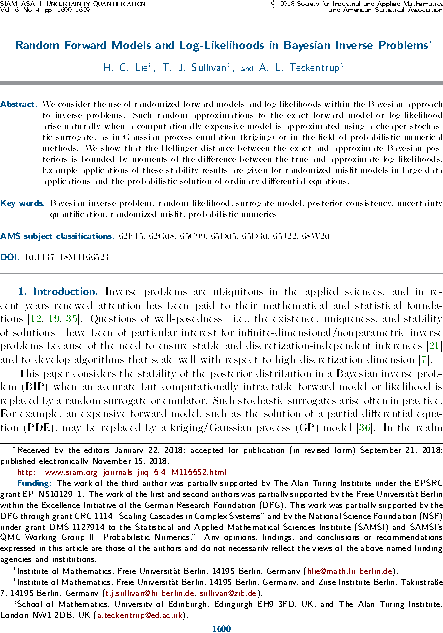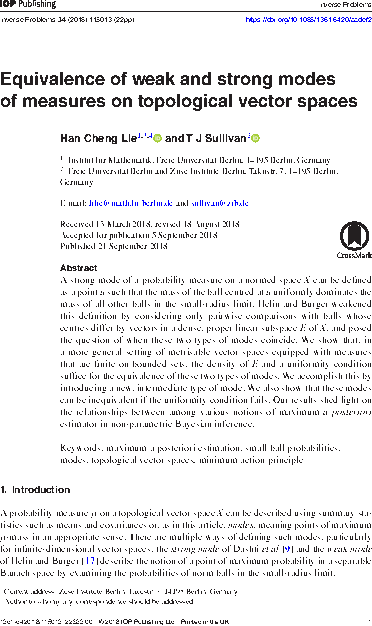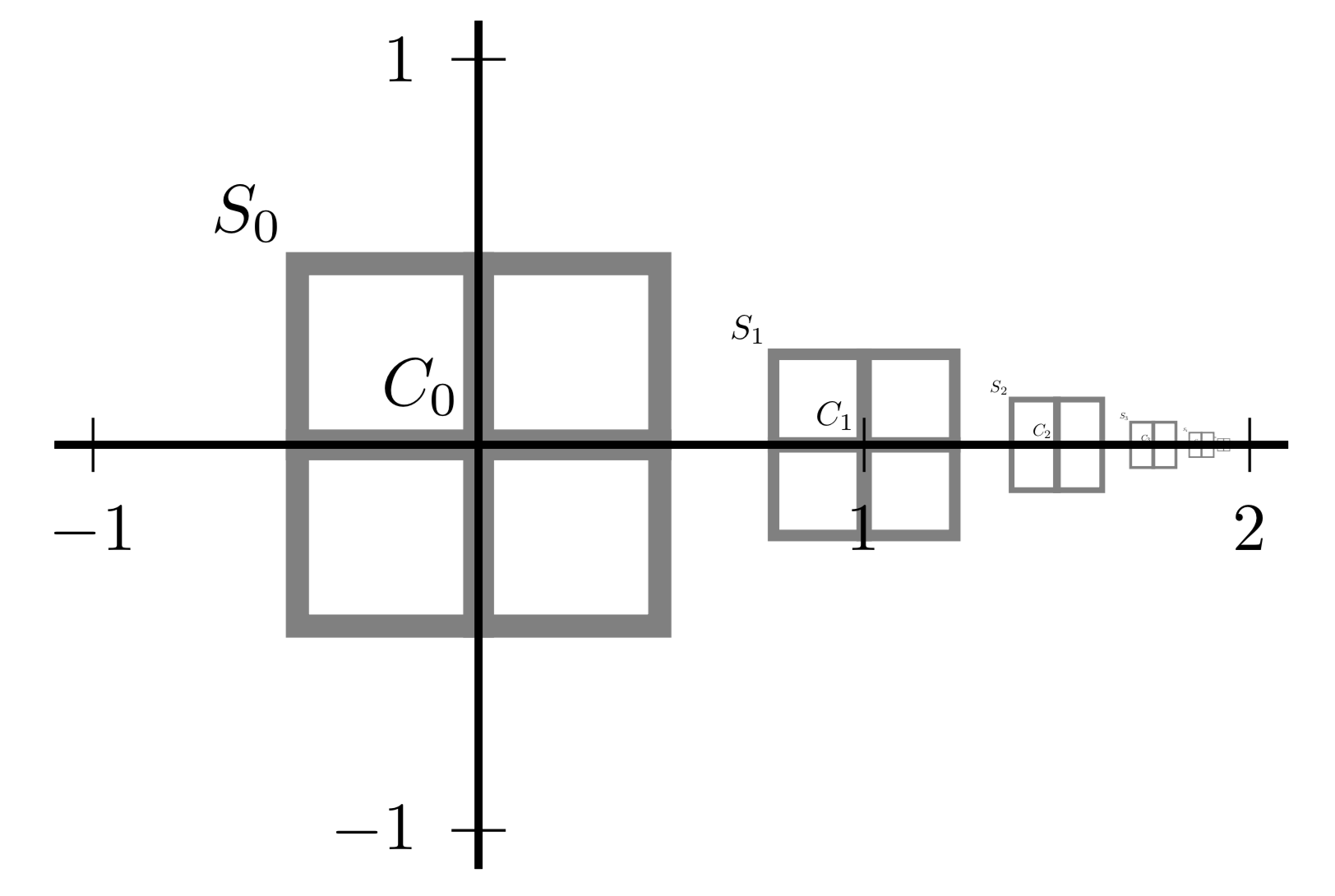# Tim Sullivan

### #lie

Clear Search### Strong convergence rates of probabilistic integrators for ODEs in Statistics and Computing

The special issue of Statistics and Computing dedicated to probabilistic numerics carries the article “Strong convergence rates of probabilistic integrators for ODEs” by Han Cheng Lie, Andrew Stuart, and myself on the convergence analysis of randomised perturbation-based time-stepping methods for the solution of ODE initial value problems.

H. C. Lie, A. M. Stuart, and T. J. Sullivan. “Strong convergence rates of probabilistic integrators for ordinary differential equations.” Statistics and Computing 29(6):1265–1283, 2019. doi:10.1007/s11222-019-09898-6

Abstract. Probabilistic integration of a continuous dynamical system is a way of systematically introducing discretisation error, at scales no larger than errors introduced by standard numerical discretisation, in order to enable thorough exploration of possible responses of the system to inputs. It is thus a potentially useful approach in a number of applications such as forward uncertainty quantification, inverse problems, and data assimilation. We extend the convergence analysis of probabilistic integrators for deterministic ordinary differential equations, as proposed by Conrad et al. (2016), to establish mean-square convergence in the uniform norm on discrete- or continuous-time solutions under relaxed regularity assumptions on the driving vector fields and their induced flows. Specifically, we show that randomised high-order integrators for globally Lipschitz flows and randomised Euler integrators for dissipative vector fields with polynomially bounded local Lipschitz constants all have the same mean-square convergence rate as their deterministic counterparts, provided that the variance of the integration noise is not of higher order than the corresponding deterministic integrator. These and similar results are proven for probabilistic integrators where the random perturbations may be state-dependent, non-Gaussian, or non-centred random variables.

Published on Tuesday 5 November 2019 at 12:00 UTC #publication #stat-comp #prob-num #lie #stuart### Implicit Probabilistic Integrators in NeurIPS

The paper “Implicit probabilistic integrators for ODEs” by Onur Teymur, Han Cheng Lie, Ben Calderhead and myself has now appeared in Advances in Neural Information Processing Systems 31 (NeurIPS 2018). This paper forms part of an expanding body of work that provides mathematical convergence analysis of probabilistic solvers for initial value problems, in this case implicit methods such as (probabilistic versions of) the multistep Adams–Moulton method.

O. Teymur, H. C. Lie, T. J. Sullivan, and B. Calderhead. “Implicit probabilistic integrators for ODEs” in Advances in Neural Information Processing Systems 31 (NIPS 2018), ed. S. Bengio, H. Wallach, H. Larochelle, K. Grauman, N. Cesa-Bianchi, and R. Garnett. 7244–7253, 2018. http://papers.nips.cc/paper/7955-implicit-probabilistic-integrators-for-odes

Abstract. We introduce a family of implicit probabilistic integrators for initial value problems (IVPs), taking as a starting point the multistep Adams–Moulton method. The implicit construction allows for dynamic feedback from the forthcoming time-step, in contrast to previous probabilistic integrators, all of which are based on explicit methods. We begin with a concise survey of the rapidly-expanding field of probabilistic ODE solvers. We then introduce our method, which builds on and adapts the work of Conrad et al. (2016) and Teymur et al. (2016), and provide a rigorous proof of its well-definedness and convergence. We discuss the problem of the calibration of such integrators and suggest one approach. We give an illustrative example highlighting the effect of the use of probabilistic integrators — including our new method — in the setting of parameter inference within an inverse problem.

Published on Thursday 13 December 2018 at 12:00 UTC #publication #nips #neurips #prob-num #lie #teymur #calderhead### Random Bayesian inverse problems in JUQ

The article “Random forward models and log-likelihoods in Bayesian inverse problems” by Han Cheng Lie, Aretha Teckentrup, and myself has now appeared in its final form in the SIAM/ASA Journal on Uncertainty Quantification, volume 6, issue 4. This paper considers the effect of approximating the likelihood in a Bayesian inverse problem by a random surrogate, as frequently happens in applications, with the aim of showing that the perturbed posterior distribution is close to the exact one in a suitable sense. This article considers general randomisation models, and thereby expands upon the previous investigations of Stuart and Teckentrup (2018) in the Gaussian setting.

H. C. Lie, T. J. Sullivan, and A. L. Teckentrup. “Random forward models and log-likelihoods in Bayesian inverse problems.” SIAM/ASA Journal on Uncertainty Quantification 6(4):1600–1629, 2018. doi:10.1137/18M1166523

Abstract. We consider the use of randomised forward models and log-likelihoods within the Bayesian approach to inverse problems. Such random approximations to the exact forward model or log-likelihood arise naturally when a computationally expensive model is approximated using a cheaper stochastic surrogate, as in Gaussian process emulation (kriging), or in the field of probabilistic numerical methods. We show that the Hellinger distance between the exact and approximate Bayesian posteriors is bounded by moments of the difference between the true and approximate log-likelihoods. Example applications of these stability results are given for randomised misfit models in large data applications and the probabilistic solution of ordinary differential equations.

Published on Monday 10 December 2018 at 12:00 UTC #publication #bayesian #inverse-problems #juq #prob-num #lie #teckentrup### Weak and strong modes in Inverse Problems

The paper “Equivalence of weak and strong modes of measures on topological vector spaces” by Han Cheng Lie and myself has now appeared in Inverse Problems. This paper addresses a natural question in the theory of modes (or maximum a posteriori estimators, in the case of posterior measure for a Bayesian inverse problem) in an infinite-dimensional space $$X$$. Such modes can be defined either strongly (a la Dashti et al. (2013), via a global maximisation) or weakly (a la Helin and Burger (2015), via a dense subspace $$E \subset X$$). The question is, when are strong and weak modes equivalent? The answer turns out to be rather subtle: under reasonable uniformity conditions, the two kinds of modes are indeed equivalent, but finite-dimensional counterexamples exist when the uniformity conditions fail.

H. C. Lie and T. J. Sullivan. “Equivalence of weak and strong modes of measures on topological vector spaces.” Inverse Problems 34(11):115013, 2018. doi:10.1088/1361-6420/aadef2

(See also H. C. Lie and T. J. Sullivan. “Erratum: Equivalence of weak and strong modes of measures on topological vector spaces (2018 Inverse Problems 34 115013).” Inverse Problems 34(12):129601, 2018. doi:10.1088/1361-6420/aae55b )

Abstract. A strong mode of a probability measure on a normed space $$X$$ can be defined as a point $$u \in X$$ such that the mass of the ball centred at $$u$$ uniformly dominates the mass of all other balls in the small-radius limit. Helin and Burger weakened this definition by considering only pairwise comparisons with balls whose centres differ by vectors in a dense, proper linear subspace $$E$$ of $$X$$, and posed the question of when these two types of modes coincide. We show that, in a more general setting of metrisable vector spaces equipped with non-atomic measures that are finite on bounded sets, the density of $$E$$ and a uniformity condition suffice for the equivalence of these two types of modes. We accomplish this by introducing a new, intermediate type of mode. We also show that these modes can be inequivalent if the uniformity condition fails. Our results shed light on the relationships between among various notions of maximum a posteriori estimator in non-parametric Bayesian inference.

Published on Saturday 22 September 2018 at 12:00 UTC #publication #inverse-problems #modes #map-estimators #lie### Weak and strong modes

Han Cheng Lie and I have just uploaded a revised preprint of our paper, “Equivalence of weak and strong modes of measures on topological vector spaces”, to the arXiv. This addresses a natural question in the theory of modes (or maximum a posteriori estimators, in the case of posterior measure for a Bayesian inverse problem) in an infinite-dimensional space $$X$$. Such modes can be defined either strongly (a la Dashti et al. (2013), via a global maximisation) or weakly (a la Helin and Burger (2015), via a dense subspace $$E \subset X$$). The question is, when are strong and weak modes equivalent? The answer turns out to be rather subtle: under reasonable uniformity conditions, the two kinds of modes are indeed equivalent, but finite-dimensional counterexamples exist when the uniformity conditions fail.

Abstract. A strong mode of a probability measure on a normed space $$X$$ can be defined as a point $$u \in X$$ such that the mass of the ball centred at $$u$$ uniformly dominates the mass of all other balls in the small-radius limit. Helin and Burger weakened this definition by considering only pairwise comparisons with balls whose centres differ by vectors in a dense, proper linear subspace $$E$$ of $$X$$, and posed the question of when these two types of modes coincide. We show that, in a more general setting of metrisable vector spaces equipped with non-atomic measures that are finite on bounded sets, the density of $$E$$ and a uniformity condition suffice for the equivalence of these two types of modes. We accomplish this by introducing a new, intermediate type of mode. We also show that these modes can be inequivalent if the uniformity condition fails. Our results shed light on the relationships between among various notions of maximum a posteriori estimator in non-parametric Bayesian inference.

Published on Monday 9 July 2018 at 08:00 UTC #preprint #inverse-problems #modes #map-estimators #lie